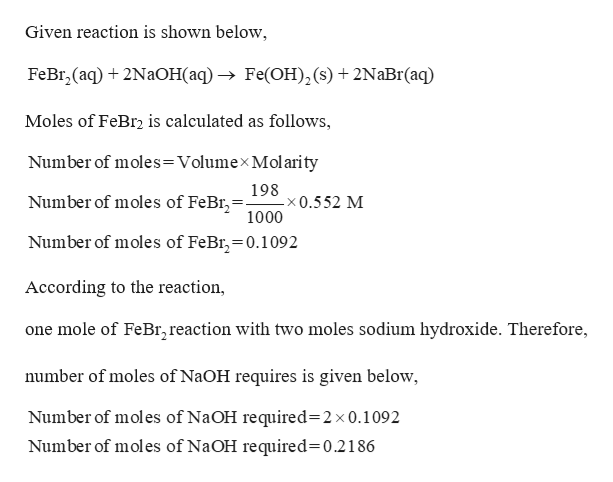Calculate the number of milliliters of 0.538 M NaOH required to precipitate all of the Fe2+ ions in 198 mL of 0.552 M FeBr2 solution as Fe(OH)2. The equation for the reaction is:FeBr2(aq) + 2NaOH(aq) Fe(OH)2(s) + 2NaBr(aq)

Question

Calculate the number of milliliters of 0.538 M NaOH required to precipitate all of the Fe2+ ions in 198 mL of 0.552 M FeBr2 solution as Fe(OH)2. The equation for the reaction is:

FeBr2(aq) + 2NaOH(aq) Fe(OH)2(s) + 2NaBr(aq)

Step 1

Given,

198 ml of 0.552 M of FeBr...help_outlineImage TranscriptioncloseGiven reaction is shown below FeBr, (aq) 2NaOH(aq) Fe(OH)2 (s) + 2NaBr(aq) Moles of FeBr2 is calculated as follows, Number of moles= Volumex Molarity 198 -x0.5 52 M 1000 Number of moles of FeBr,- Number of moles of FeBr, 0.1092 According to the reaction, one mole of FeB,reaction with two moles sodium hydroxide. Therefore, number of moles of N2OH requires is given below, Number of moles of NaOH required=2x0.1092 Number of moles of NaOH required 0.2186 fullscreen

Want to see the full answer?

See Solution

Want to see this answer and more?

Our solutions are written by experts, many with advanced degrees, and available 24/7

See Solution
Tagged in

General Chemistry